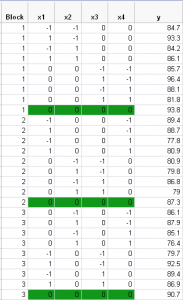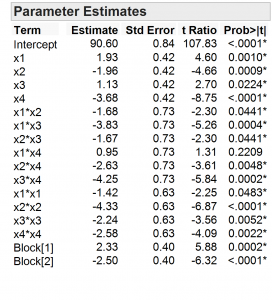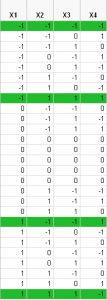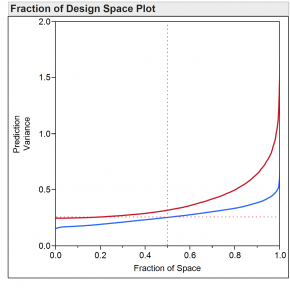Choose Language Hide Translation Bar
Celebrating George Box and Box-Behnken designs

As part of the International Year of Statistics, the JMP Blog is honoring influential statisticians each month. Professor George E.P. Box is the honoree for May. Last week, I wrote about on the first of his two-part paper with J. Stuart Hunter on the family of regular two-level fractional factorial designs that was published in Technometrics in 1961.

In this post, I focus on the famous Box-Behnken designs, which are very popular designs for fitting quadratic response surfaces. Box-Behnken designs are notable in that each factor is restricted to three levels – just enough to allow for fitting a quadratic term in each factor. Another notable feature of these designs is that each run other than the center runs has at least one factor set to zero in scaled units. This means that there are no runs where every factor is at one of its extreme values. This is in stark contrast to the regular two-level fractional factorial designs where every run has every factor at either -1 or +1 in scaled units.

How does the paper begin?

The paper starts by pointing out that quantitative factors could be set to a theoretically infinite number values. Though they admit that there is no “essential need to restrict” to a few levels, they argue that convenience requires the use of just a few levels.

They go on to introduce the concept of a “redundancy factor,” which is the fraction by which the number of runs in a design exceeds the number of parameters in the model of interest. They point out that the number of parameters in a polynomial of degree, d, in k factors is (k+d)!/(k!d!). In general, a full-factorial design has substantially more runs than necessary to fit the required number of terms. They point out that using the three-level full factorial design for five factors for fitting a full quadratic model requires 243 runs. But this model only has 21 unknown parameters to estimate. So, the full-factorial design has more than 11 times as many runs than are needed. They conclude: “In situations in which the experimental error variance is not so large as to require large numbers of observations to obtain necessary precision, designs having small redundancy factors are desirable.” I could not agree more!

The ability to keep the redundancy factor small while providing a design that allows for fitting a full quadratic model provides the motivation for their new class of designs.

What happens next?

Having motivated the need for a new family of designs having three levels for each factor and capable of fitting a full quadratic model, they turn to the clever design construction idea that results in their new class of designs. Their idea was to combine two-level factorial designs with balanced incomplete block designs “in a particular manner.”

A Box-Behnken design has groups of runs where for each run in the group only a certain number of factors change. For this group of runs, all the other factors are set at zero in scaled units. The identity of the factors that vary in each group of runs changes from one group to the next. For example, in the first group of runs, x1 and x2 might be varied, and in the next group of runs, x3 and x4 might be the variable factors.

The complete pattern of these changes is described by a balanced incomplete block design. These designs have the property that every treatment occurs the same number of times and every treatment occurs in a block with every other treatment the same number of times. In the Box-Behnken design, the “treatments” are the varying factors in a group. The blocks are how many factors are allowed to vary in a group.

Can you show an example?

Figure 1 shows a Box-Behnken design for four factors that appears as Table 6 in their paper. The first column is the Block column, which shows how to block the Box-Behnken design into three orthogonal blocks. Each block contains two groups of four runs plus a center run.Note that in the first group x1 and x2 vary. In the second group, x3 and x4 vary. In the third group (second block)  x1 and x4 vary. In the fourth group, x2 and x3 vary. In the fifth group, x2 and x4 vary, and in the last group, x1 and x3 vary. Each factor varies in three groups, and each factor varies once in combination with every other factor. This pattern of the varying factors matches a balanced incomplete block plan with four treatments (the factor identities) and six blocks (the groups of runs), where there are two runs per block. The two “runs” are the two factors that vary in the groups.

The groups of runs are a 2x2 full factorial in the two factors that are varying.

How does the rest of the paper go?

After introducing the basic idea, they show how to block these designs orthogonally and how to add center runs to reduce the prediction variance in the center of the design region. They give examples of their family of designs for 3- 7, 9-12 and 16 factors. The designs for more than seven factors require substantially more than 100 runs and do not appear as options in the Response Surface design options in JMP.

After introducing the designs, they also show how to analyze data generated using these designs. This includes providing the estimates of the coefficients with their accompanying standard errors. This part of the paper does not use the matrix formulation for finding the least squares estimates and their standard errors. The four-factor case shown in Figure 1 has 15 coefficients to estimate – the intercept, four linear effects, six two-factor interaction effects and four quadratic effects. The modern approach is to create the model matrix, X, having 15 columns (one for each coefficient) and as many rows as there are runs in the design. The part that computers of 1960 could not easily do was to find the inverse of the matrix, X’X, which is required for both computing the coefficient estimates and their standard errors. To avoid this computation complication, they provide a table of constants for each design and a computational approach using these constants and various sums of products of the y’s  and the x’s.

Figure 2 shows the parameter estimates and their associated standard errors for the table in Figure 1. All the coefficients match those in the paper. However, the paper does not calculate the standard error of the quadratic effects for the four-factor example correctly. So, the paper reports these standard errors as .66 when the correct value is .63Have Box-Behnken designs stood the test of time?

Box-Behnken designs are still very popular. I would recommend the three- and four-factor designs with a few caveats.

It is important to remember that these designs do not run tests at the extremes of every factor. So, predictions at these points are actually extrapolations outside the region of experimentation. Box-Behnken designs are more properly thought of as designs on a sphere rather than designs on a cube. However, I suspect that many practitioners actually use these designs to predict while allowing every factor to vary between -1 and 1 in scaled units. That is, they are thinking of this design region as cubic.

Figure 3 shows the settings of an I-optimal design for 4 factors in 27 runs – the same number as are in the Box-Behnken design in Figure 1.Note that four of the runs have all four factors at their extreme settings. There are also five center runs. Only two of the runs (other than the center runs) have more than one factor at the 0 value in scaled units.

Figure 4 shows the Fraction of the Design Space Plot for the Box-Behnken design. The red curve shows the relative prediction variance for the Box-Behnken design, and the blue curve shows the relative prediction variance for the I-optimal design.The maximum prediction variance for the Box-Behnken design is 2.3333σ2 and each vertex of the factor space has this variance. For the I-optimal design, the maximum prediction variance is 0.832647σ2 at the factor setting [1, 1, 1, 1] among others. The average variance of prediction over the cube for the Box-Behnken design is 0.4σ2. For the I-optimal design, it is 0.273σ2. It is pretty clear that if you want to be able to predict over the entire cube, the Box-Behnken design is being dramatically outperformed by the I-optimal design.

On the other hand, if you restrict yourself to predictions inside a sphere with a squared radius of 2, then the Box-Behnken design is very efficient. One final note is that even if you consider region of interest for the Box-Behnken design to be cubic, this design is relatively resistant to prediction bias due to active third-order effects.

References

Box, G. E. P. and Hunter, J. S. (1961) "The 2k-p Fractional Factorial Designs Part I" Technometrics Vol 3, No. 3 311-351.

Box, G.E.P. & Behnken, D.W. (1960). Some new three level designs for the study of quantitative variables. Technometrics 2, pp. 455-475.

Article Labels

There are no labels assigned to this post.

1 Comment
Visitor

Celebrating statisticians: Designed experiments for nonlinear models wrote:

[...] Box, as part of the celebration of the International Year of Statistics. Last week, I wrote about Box-Behnken designs for fitting response surface models. In this post, I want to tell you about the paper Box wrote in [...]